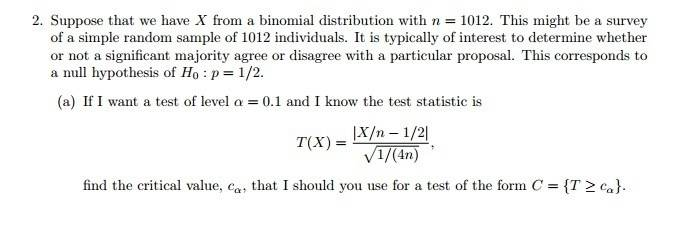# Using approximations to the binomial distribution

## Homework Statement

This is the problem I am given.. It is in he picture below or in the thumbnail. I was also told that since ##n## is big enough that I can use normal approximations.

## The Attempt at a Solution

I think that ##C_{\alpha}=C_{0.1}=2.33## which I got off the Z-score chart. The test statistic given looks like the one given for a binomial distribution given by where ##Z=\dfrac{x-np}{\sqrt{npq}}=\dfrac{\frac{x}{n}-p}{\frac{\sqrt{pq}}{\sqrt{n}}}##. I am not sure if this right or not. But it seems like this is the only way of finding the critical value. Thanks[/B]

Last edited: